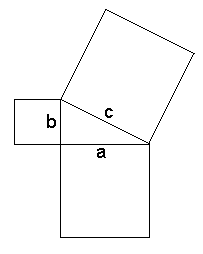Tuesday, September 6

• More Introduction to course organization.
• Web Materials and Notes.
• What is Visual Mathematics?
• Mathematics that studies topics related to visual experience. [Geometry, Topology, Motion]
• Visualization of mathematics that is not inherently visual. [Visualizing Counting]
• Example: Numbers...
• numeral: a symbol for representing a number
• Number: a form of universal language to describe anything/ physical things/ concepts related to measurement
• such as V, 5, five, cinq, chamesh, cinco
• Frege distinguished numerals from numbers in the late 19th century.
• We can compare numbers... for instance we say" 3 is less than 5"
• Is 3 smaller than 5?
• Numerals are symbols (visual or linguistic) that we use to represent numbers.
• We use numbers to measure (lengths) and put things in order (which was first).
• Another common visual representaion of numbers uses the number line.
• ___.___.___.___.___.___.___.______
•       1     2     3     4     5     6     7

• Here the numerals are associated with points, so the points are considered to visualize the corresponding numbers.

[Use Wingeometry to illustrate numbers used for measurement.]

• We visualize equations that give relations between numbers with graphs in the coordinate plane.

• 3x + 2y = 6 is visualized by the graph of a line.
Use Wingeometry to visualize coordinates, points, and lines.

• Miscellaneous: Some topics we will study.
• The film lists as a guide to the course topics.
• Symmetry.(Chapter 5)
• Tiling. (Chapter 5)
• Similarity and Projection. (Chapter 4)
• The color problem. (Chapter 6)
• The motion problem.(Chapter 3)
• The Sphere and the Torus. Surfaces (Chapter 6)

Theme Question:Who first showed the earth was a sphere?

• Discussion (brief) on the The Sphere  and the Torus.
• Coop work- 3-4 partners- record responses of group. 5 minutes, followed by discussion.

Question 1. How can one distinguish the sphere from a plane (Flatland) based solely on experiences on the surface?

Question 2. How can one distinguish the sphere from a torus based solely on experiences on the surface?

• Measurement and the Pythagorean Theorem (PT)
•a2 + b2 = c2

Show video on PT.
Outline:
Area of triangles = 1/2 bh
Area of parallelogram= bh
Scaling: a linear scale change of r gives area change of factor r2.
3 questions: running, moat, wind power...
Proof of the PT: Similar right triangles: c= a2 /c + b2 /c .
applications and other proofs.
Prop. 47 of Euclid.
Dissection Proof.
Prop 31 Book  VI  Similar shapes.
Simple proof of PT using similar triangles of the triangle.
Use in 3 dimensional space